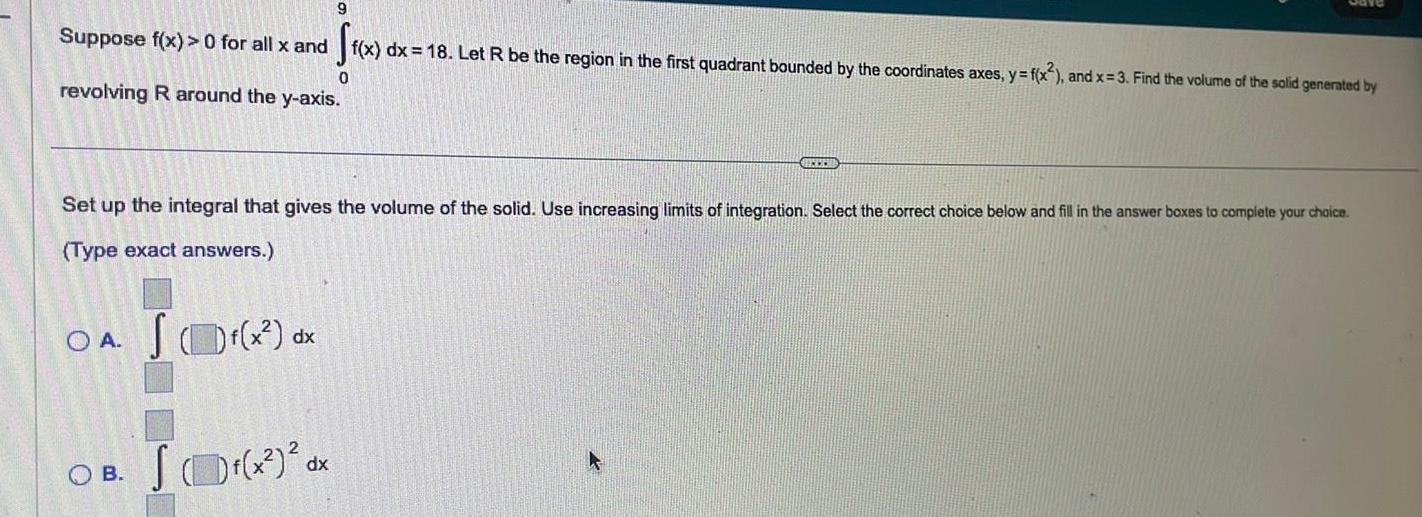Question:

# 9 Suppose f x 0 for all x and f x dx 18 Let R be the region

Last updated: 11/19/20239 Suppose f x 0 for all x and f x dx 18 Let R be the region in the first quadrant bounded by the coordinates axes y f x and x 3 Find the volume of the solid generated by 0 revolving R around the y axis BELEM Set up the integral that gives the volume of the solid Use increasing limits of integration Select the correct choice below and fill in the answer boxes to complete your choice Type exact answers OA JOf x dx OB SD x dx